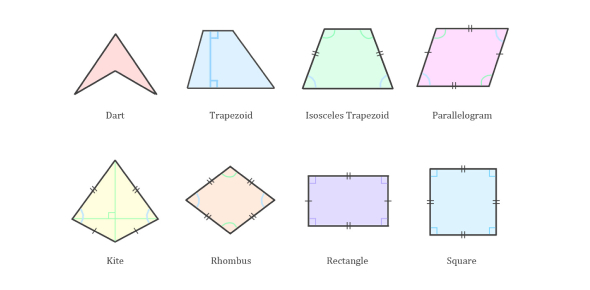5 Questions | Total Attempts: 79Settings• 1.
Explain your answer to question 4. Which one of these did you chose and why? Rectangles, Parallelograms, Quadrilaterals, or Squares.
• 2.
A quadrilateral with 2 pairs of parallel sides is always a parallelogram.
• A.

True

• B.

False

• 3.
Rhombi, Rectangles, and Squares are special types of _________________.
• 4.
A square has its own properties and all the properties of ____________(select all that are correct)
• A.

Circles

• B.

Rectangles

• C.

Triangles

• D.

Rhombi

• E.

Parallelograms

• 5.
In class we have talked about the "quadrilateral family tree". Which of the following is at the top of the tree?
• A.

Rectangles

• B.

Parallelograms

• C.Back to top